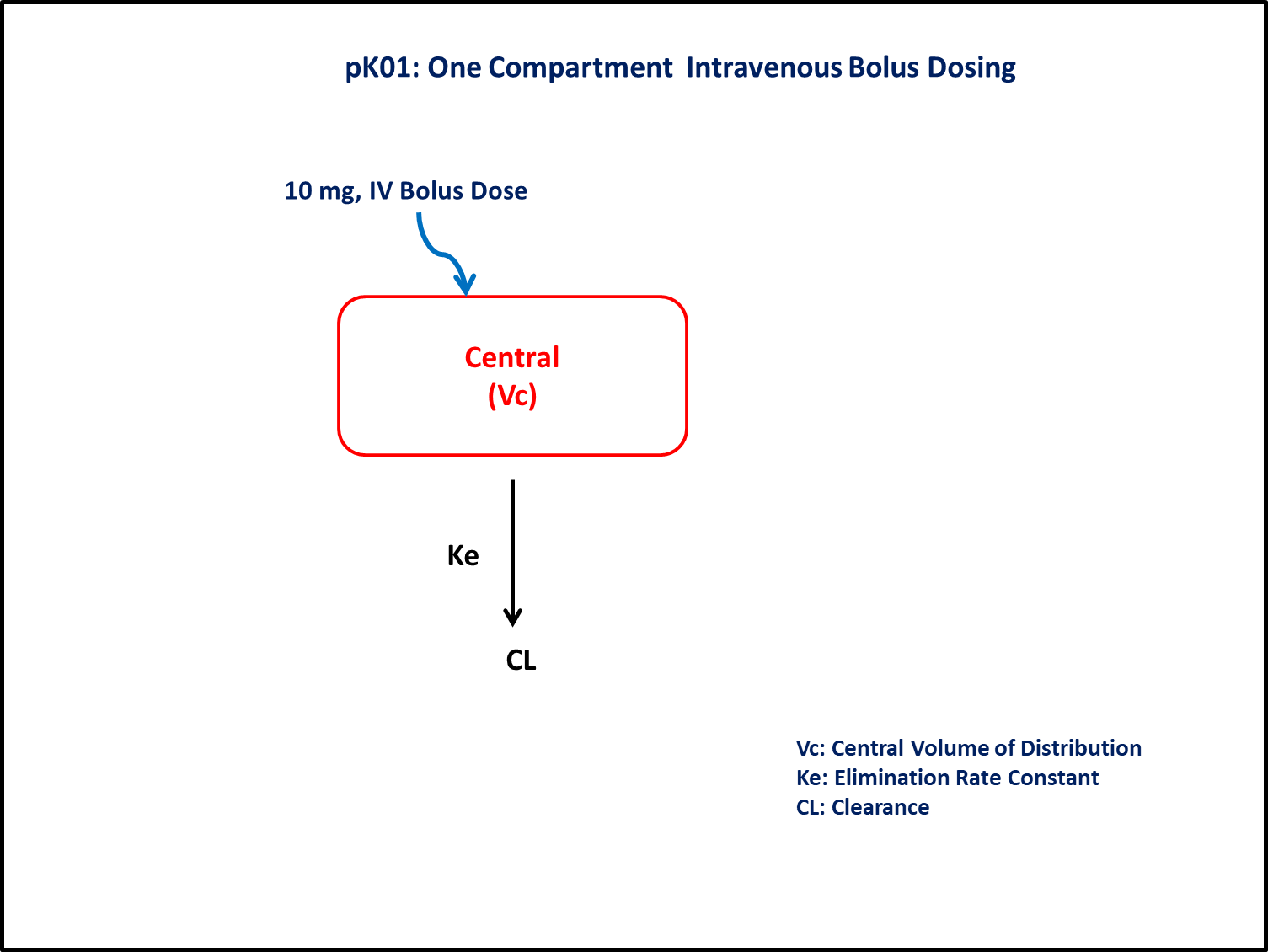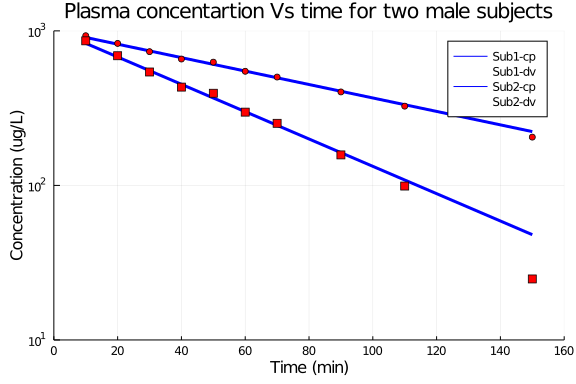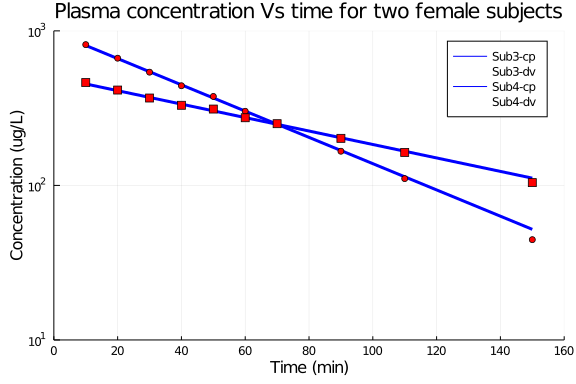# Exercise PK01 - One Compartment Intravenous Bolus Dosing

### Background

• Structural model - One compartment linear elimination

• Route of administration - IV bolus

• Dosage Regimen - 10 mg IV

• Number of Subjects - 4### Learning Outcome

In this model, you will learn -

• To build One compartment model for four subjects given Intravenous Bolus dosage.

• To estimate the fundamental parameters involved in building the model.

• To apply differential equation in the model as per the compartment model.

• To design the dosage regimen for the subjects and simulate the plot.

### Objectives

In this tutorial, you will learn how to build one compartment model and to simulate the model for four subjects with different values of Parameter estimates.

### Libraries

call the "necessary" libraries to get started.

using Pumas
using Plots
using CSV
using StatsPlots
using Random


### Model

In this One compartment model, intravenous dose is administered into the central compartment. We account for rate of change of concentration of drug in plasma (Central Compartment) for the time duration upto 150 min.

pk_01        = @model begin
@param begin
tvcl     ∈ RealDomain(lower=0)
tvvc     ∈ RealDomain(lower=0)
Ω        ∈ PDiagDomain(2)
σ        ∈ RealDomain(lower=0)
end

@random begin
η        ~ MvNormal(Ω)
end

@pre begin
Cl       = tvcl * exp(η)
Vc       = tvvc * exp(η)
end

@dynamics begin
Central' = -(Cl/Vc)*Central
end

@derived begin
cp       = @. 1000*(Central/Vc)
dv       ~ @. Normal(cp, σ)
end
end

PumasModel
Parameters: tvcl, tvvc, Ω, σ
Random effects: η
Covariates:
Dynamical variables: Central
Derived: cp, dv
Observed: cp, dv


### Parameters

In this exercise, parameter estimate values for each subject are different. For each subject, parameters are defined individually wherein tv represents the typical value for parameters. Parameters provided for simulation are:-

• Cl - Clearance(L/hr),

• Vc - Volume of Central Compartment(L),

• Ω - Between Subject Variability,

• σ - Residual error

param1 = (tvcl = 0.10,
tvvc = 9.98,
Ω    = Diagonal([0.00,0.00]),
σ    = 20.80)

(tvcl = 0.1, tvvc = 9.98, Ω = [0.0 0.0; 0.0 0.0], σ = 20.8)

param2 = (tvcl = 0.20,
tvvc = 9.82,
Ω    = Diagonal([0.00,0.00]),
σ    = 27.46)

(tvcl = 0.2, tvvc = 9.82, Ω = [0.0 0.0; 0.0 0.0], σ = 27.46)

param3 = (tvcl = 0.20,
tvvc = 10.22,
Ω    = Diagonal([0.00,0.00]),
σ    = 8.78)

(tvcl = 0.2, tvvc = 10.22, Ω = [0.0 0.0; 0.0 0.0], σ = 8.78)

param4 = (tvcl = 0.20,
tvvc = 19.95,
Ω    = Diagonal([0.00,0.00]),
σ    = 8.50)

(tvcl = 0.2, tvvc = 19.95, Ω = [0.0 0.0; 0.0 0.0], σ = 8.5)


### Dosage Regimen

10 mg IV bolus dosage administered to four subjects at time zero.

Note:- The concentrations are in μg/L and dose is in mg, thus the final conc is multiplied by 1000 in the model

ev1   = DosageRegimen(10,time=0,cmt=1)
sub1  = Subject(id=1,events=ev1)
sub2  = Subject(id=2,events=ev1)
sub3  = Subject(id=3,events=ev1)
sub4  = Subject(id=4,events=ev1)


### Simulation

• Lets simulate for plasma concentration for four subjects for specific observation time points after IV bolus dose.

Random.seed!(123)
sim_s1 = simobs(pk_01, sub1, param1, obstimes=[10,20,30,40,50,60,70,90,110,150])
Random.seed!(123)
sim_s2 = simobs(pk_01, sub2, param2, obstimes=[10,20,30,40,50,60,70,90,110,150])
Random.seed!(123)
sim_s3 = simobs(pk_01, sub3, param3, obstimes=[10,20,30,40,50,60,70,90,110,150])
Random.seed!(123)
sim_s4 = simobs(pk_01, sub4, param4, obstimes=[10,20,30,40,50,60,70,90,110,150]);


### DataFrame & Plots

• cp - Predicted concentration

• dv - Observed concentration

• sub1,sub2,sub3,sub4 - corresponds to subject id for four subjects

#### For two male subjects

df1 = DataFrame(sim_s1)
df2 = DataFrame(sim_s2)
@df df1 plot(:time, :cp, yaxis=:log,
label="Sub1-cp",xlabel="Time (min)",ylabel="Concentration (ug/L)",
title="Plasma concentartion Vs time for two male subjects",color=[:blue], linewidth=3,
xlims=(0,160), xticks=[0,20,40,60,80,100,120,140,160], ylims=(10,1000),yticks=[10,100,1000])
@df df1 scatter!(:time, :dv,
label="Sub1-dv",color=[:red], markershape=[:circle])
@df df2 plot!(:time, [:cp], yaxis=:log,
label="Sub2-cp", color=[:blue], linewidth=3)
@df df2 scatter!(:time, :dv,
label="Sub2-dv",color=[:red], markershape=[:square])#### For two female subjects

df3 = DataFrame(sim_s3)
df4 = DataFrame(sim_s4)
@df df3 plot(:time, :cp, yaxis=:log,
label="Sub3-cp", xlabel="Time (min)", ylabel="Concentration (ug/L)",
title="Plasma concentration Vs time for two female subjects", color=[:blue],linewidth=3,
xlims=(0,160), xticks=[0,20,40,60,80,100,120,140,160], ylims=(10,1000), yticks=[10,100,1000])
@df df3 scatter!(:time, :dv,
label="Sub3-dv", color=[:red], markershape=[:circle])
@df df4 plot!(:time, :cp, yaxis=:log,
label="Sub4-cp", color=[:blue], linewidth=3)
@df df4 scatter!(:time, :dv,
label="Sub4-dv", color=[:red], markershape=[:square])